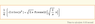# Integrate x^2 sin(x^2) dx

jacy
Hi ,
I am having trouble integrating this problem.

Integrate x^2 sin(x^2) dx

Here is what i did. I used the substitution method.

u = x^2 sqrt u = x
du = 2x dx
du/2x = dx

du/2 sqrt u = dx since sqrt u = x

substituting this in the equation

u sin(u) du/2 sqrt u

Townsend
jacy said:
Hi ,
I am having trouble integrating this problem.

Integrate x^2 sin(x^2) dx

I don't think the antiderivative of your integrand is an elementary...but I don't really know for sure.

amcavoy
I believe you're correct. My computer cannot calculate it.

jacy
apmcavoy said:
I believe you're correct. My computer cannot calculate it.

Thanks 4 ur time. Do u think that problem is wrong.

Townsend
jacy said:
Thanks 4 ur time. Do u think that problem is wrong.

The problem is fine...it's just that you cannot find an elementary antiderivative as a solution.

Homework Helper
where'd you obtain the problem?

amcavoy
It would work if the integrand was $x\sin{x^{2}}$.

jacy
GCT said:
where'd you obtain the problem?

Thanks for looking at the problem. My teacher gave this problem on the exam. How can we solve this.

Tony11235
Integration by parts might be possible, but my computer and my TI 89 cannot compute it, so there's probably not a simple antiderivative. And you were given this problem on a test?jacy
Tony11235 said:
Integration by parts might be possible, but my computer and my TI 89 cannot compute it, so there's probably not a simple antiderivative. And you were given this problem on a test?Thanks, do u think the substitution method that i used will work. This wasn't the only one there were 3 more.

Last edited:
amcavoy
Are you sure it wasn't asking you to evaluate it as a definite integral numerically? That's the only way I can see doing this.

jacy
apmcavoy said:
Are you sure it wasn't asking you to evaluate it as a definite integral numerically? That's the only way I can see doing this.

No it isn't a definite integral. It's tough one. Hopefully the teacher should provide the solution today. If he does, then can i post the solution in here, thanks.

whozum
$\int x^2\sin(x^2) dx [/tex] [itex] \int x^2\sin(x^2) dx = \frac{-x}{2}\cos(x^2) + \frac{1}{2} \int \cos(x^2) dx [/tex] Staff Emeritus Science Advisor Gold Member whozum said: [itex] \int x^2\sin(x^2) dx [/tex] [itex] \int x^2\sin(x^2) dx = \frac{-x}{2}\cos(x^2) + \frac{1}{2} \int \cos(x^2) dx [/tex] True, but now what's [itex]\frac{1}{2} \int \cos(x^2) dx$?

jacy, the initial response you received was correct. Your integrand doesn't have an elementary antiderivative. 10 bucks says that the problem was supposed to be $\int x\sin(x^2) dx$.

whozum
Tom Mattson said:
True, but now what's $\frac{1}{2} \int \cos(x^2) dx$?

jacy, the initial response you received was correct. Your integrand doesn't have an elementary antiderivative. 10 bucks says that the problem was supposed to be $\int x\sin(x^2) dx$.

Its not elementary i was just trying it out and seeing how far I could get, but figured I'd just post it anyway.

Gold Member
MHB
Here's the answer from Mathematica in case anyone was wondering. I agree, it was probably supposed to be $$\int x\sin{(x^2)}dx$$.

#### Attachments

•Integrate.gif
1.4 KB · Views: 502
jacy
Tom Mattson said:
True, but now what's $\frac{1}{2} \int \cos(x^2) dx$?

jacy, the initial response you received was correct. Your integrand doesn't have an elementary antiderivative. 10 bucks says that the problem was supposed to be $\int x\sin(x^2) dx$.

I don't think so it was a typo. This problem was on the exam, thanks.

whozum
jacy said:
I don't think so it was a typo. This problem was on the exam, thanks.

Was the problem asking to find the derivative of that function?

jacy
whozum said:
Was the problem asking to find the derivative of that function?

We have to find the anti derivative of that function. Today the teacher said that he made a typo it should be
integrate x^2 sin^2(x) instead of x^2 sin(x^2)

How did u guys type the sign of integral

bomba923
$$\int {x^2 \sin ^2 x\,dx}$$
can be evaluated via integration by parts.

jacy, the integral sign is just \int when using LaTeX.
Try clicking on this integral sign: $$\int$$

jacy
bomba923 said:
$$\int {x^2 \sin ^2 x\,dx}$$
can be evaluated via integration by parts.

jacy, the integral sign is just \int when using LaTeX.
Try clicking on this integral sign: $$\int$$

Thanks, yea now i can solve it